## A light-rail train going from one station to the next on a straight section of track accelerates from rest at 1.1 m/s^2 for 20s. It then pro

Question

A light-rail train going from one station to the next on a straight section of track accelerates from rest at 1.1 m/s^2 for 20s. It then proceeds at constant speed for 1100m before slowing down at 2.2m/s^2 until it stops at the station. A) What is the distance between stations? B) How much time does it take the train to go between the stations?

in progress 0
6 months 2021-07-30T04:19:54+00:00 1 Answers 11 views 0

A) The distance between the stations is 1430m

B) The time it takes the train to go between the stations is 80s

Explanation:

First we will calculate the distance covered for the first 20s.

From one the equations of kinematics for linear motion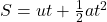Where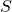is distance traveled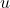is the initial velocityis time

and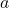is acceleration

Since the train starts from rest,= 0 m/s

Hence,  for the first 20s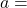1.1 m/s²;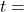20s,= 0 m/sgives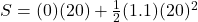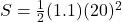220m

This is the distance covered in the first 20s.

• The train then proceeds at constant speed for 1100m.

Now, we will calculate the speed attained here

From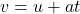Where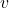is the final velocity

Hence,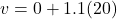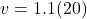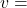22 m/s

This is the constant speed attained when it proceeds for 1100m

• The train then slows down at a rate of 2.2 m/s² until it stops

We can calculate the distance covered while slowing down from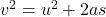The initial velocity,here will be the final velocity before it started slowing down= 22 m/s

The final velocity will be 0, since it came to a stop.= 0 m/s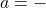2.2 m/s² ( – indicates deceleration)

Hence,gives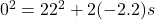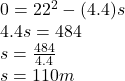This is the distance traveled while slowing down.

A) The distance between the stations is

220m + 1100m + 110m

= 1430m

Hence, the distance between the stations is 1430m

B) The time it takes the train to go between the stations

The time spent while accelerating at 1.1 m/s² is 20s

We will calculate the time spent when it proceeds at a constant speed of 22 m/s for 1100m,

From,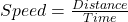Then,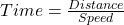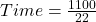Time = 50 s

And then, the time spent while decelerating (that is, while slowing down)

From,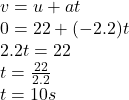This is the time spent while slowing down until it stops at the station.

Hence, The time it takes the train to go between the stations is

20s + 50s + 10s = 80s

The time it takes the train to go between the stations is 80s